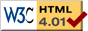# The Australian Tax File Number

A TFN is a number issued to a person by the Commissioner of Taxation and is used to verify client identity and establish their income levels. It is a eight or nine digit number without any embedded meaning.

This form requires JavaScript !

The program that does the TFN verification requires JavaScript. If your browser is able to understand JavaScript programs, please turn the options on in the preferences. Otherwise, you won't be able to use this page.

You can use the form below to test if a TFN is valid.

Tax File Number:

If you don't have a TFN, or if you don't want to enter yours, you can use the following number to test the system: 123 456 782.

## How does it work ?

The verification algorithm embedded in the Tax File Number is known as a check digit algorithm: one of the digits (the last one in this case) depends upon the others, meaning that if one digit is changed, the last digit will not be correct anymore.

The general procedure is as follows: each digit of the Tax File Number is multiplied by a given weight (different weights are used for the different digits), and the sum of these products should be a multiple of 11. If it is not the case, there is an error in your number.

Here is a complete example for the number 123 456 782: we multiply each digit by the weights given below:

 1 2 3 4 5 6 7 8 2 x x x x x x x x x 1 4 3 7 5 8 6 9 10 = = = = = = = = = 1 + 8 + 9 + 28 + 25 + 48 + 42 + 72 + 20 = 253

We then calculate the sum 1 + 8 + 9 + 28 + 25 + 48 + 42 + 72 + 20 = 253. Since 253 = 11 * 23, it is a multiple of 11, hence the number is valid.

## Background and sources

To the best of our knowledge, the "TFN algorithm" (the algorithm that computes the check digit described above) has not been publicly disclosed yet. When it was first designed, in the early 1970, the algorithm was kept secure within the ATO, but since then, the algorithm has been communicated to more than 20,000 external entities in Australia, such as employers, investment bodies, software makers, etc, and each of these entities had to sign a confidentiality agreement to make sure that the algorithm was kept secure.

However, as the Australian National Audit Office (ANAO) mentioned in a report, "the check digit routine used to generate the TFNs is widely known and could be regarded as in the public domain. It is one of a wide family of similar routines used throughout the world and known as check digit routines. A competent mathematician provided with a handful of numbers could with relative ease derive the TFN check digit formula".

The algorithm described above has been derived using only the information given in the report. The handful of numbers were provided (involuntarily) by the ATO itself, thanks to the e-tax program that allows anyone to easily check if a TFN is valid, and to generate TFN by trial and error.

References:Last modified: Thu Dec 11 19:11:42 EST 2003